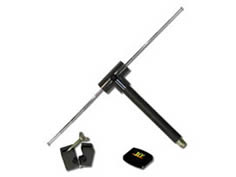Frequency and Wavelength Calculation

This frequency to wavelength calculator is especially useful for the calculation of the element length of the dipole antennas. Many of our dipole antennas have adjustable element lengths and by entering the frequency of interest this calculator will calculate the wavelength (or element length) corresponding to the required frequency. You can also enter in the wavelength and this calculator will calculate the corresponding frequency.

 Frequency Wavelength Calculator Wavelength Calculator Frequency Hz KHz MHz GHz full wave 3/4 wave 5/8 wave 1/2 wave 1/4 wave 1/8 wave Wavelength Inches Feet mm cm m KmFreq is the Frequency in cycles per second
C is the velocity factor 299,792,458 meters per second
λ represents wavelength in meters

Other useful RF Conversions:
Useful formulas for RF related conversions
Antenna Factor and Gain Calculations
dBm to Volts to Watts conversion
Magnetic field conversions
Field Intensity Calculation
Antenna Beamwidth Coverage Calculations
VSWR Calculations

Contact Details

A.H. Systems, inc.
9710 Cozycroft Ave.
Chatsworth, CA 91311

P: (818) 998-0223

F: (818) 998-6892

E: Email

Product Showcase

Dipole Antenna:• 325 MHz to 1000 MHz
• Low Insertion Loss & VSWR
• Transmit and recieve

Designed per the FCC specifications, this Tuned Dipole antenna is ideal for site attenuation measurements.